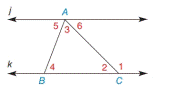Chapter 2.4, Problem 14EElementary Geometry For College St...

7th Edition
Alexander + 2 others
ISBN: 9781337614085

Solutions

Chapter
SectionElementary Geometry For College St...

7th Edition
Alexander + 2 others
ISBN: 9781337614085
Textbook Problem

In Exercises 13 to 15, j ∥ k and Δ A B C . Given: m ∠ 3 = 55 ° m ∠ 2 = 74 ° Find: m ∠ 1 , m ∠ 4 , and m ∠ 5To determine

To find:

The measure of angles m1, m4, and m5.

Explanation

Given:

In ΔABC,

m3=55°m2=74°jk

Figure (1)

Properties used:

(1) In a triangle, the sum of the measures of all interior angles is 180°.

(2) If two parallel lines are cut by a transversal, then pairs of alternate interior angles are congruent.

(3) The measure of an exterior angle of a triangle equals the sum of the measures of the two nonadjacent interior angles.

Calculation:

In ΔABC,

m3=55°m2=74°jk

In ΔABC, the sum of the measures of the interior angles is 180°.

m3+m4+m2=180°

Substitute 55° for m3 and 74° for m2 in the above equation.

Still sussing out bartleby?

Check out a sample textbook solution.

See a sample solution

The Solution to Your Study Problems

Bartleby provides explanations to thousands of textbook problems written by our experts, many with advanced degrees!

Get Started